# The 15 Basic Excel Formulas Everyone Needs to Know

Learn how to add arithmetic, string, time series, and complex formulas in Microsoft Excel.
Sep 2022  · 15 min read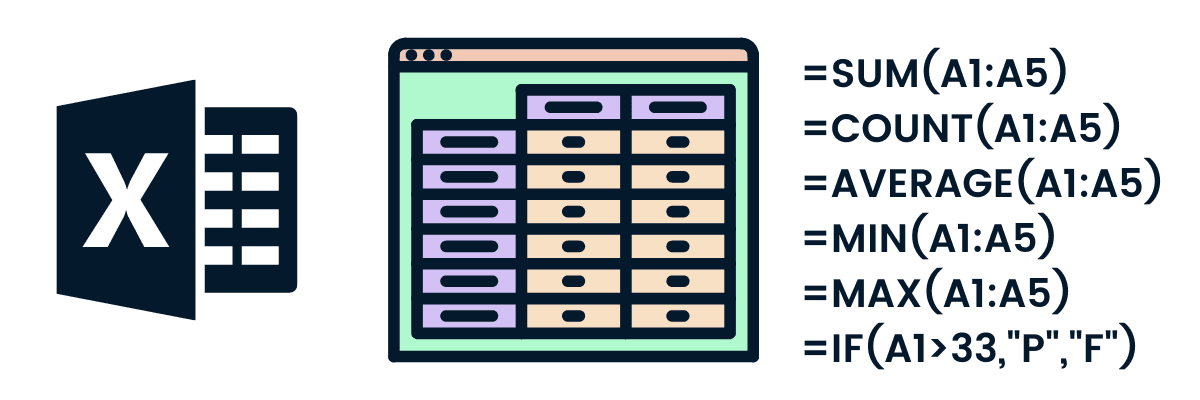## What is an Excel Formula?

Microsoft Excel is a popular tool for managing data and performing data analysis. It is used for generating analytical reports, business insights, and storing operational records. To perform simple calculations or analyses on data, we need Excel formulas.

Even simple Excel formulas allow us to manipulate string, number, and date data fields. Furthermore, you can use if-else statements, find and replace, mathematics and trigonometry, finance, logical, and engineering formulas.

Unlike programming languages, you will be writing the formula name and arguments. That’s it, nothing complex. You can also use Excel-assisted user interference to add formulas.

## How to Use Excel Formulas

Adding the Excel formula is relatively easy. It will come to you naturally if you are familiar with any business intelligence software.

The most effective and fast way to use formulas is by adding them manually. In the example below, we are calculating the BMI (Body Mass Index) of the athletes shown in the table.

BMI = weight (KG)/ (Height (m))2

1. Choose the cell for the resulting output. You can use the mouse to select the cell or use the arrow key to navigate.
2. Type “=” in the cell. The equal sign will appear in the cell and formula bar.
3. Type the address of the cell that we want to use for our calculation. In our case, it is E2 (weight/KG).
5. To convert height from centimeters to a meter, we will divide the D2 by 100.
6. Take the squared “^2” of the height and press Enter.

Note: To get the address of any cell, you need to look at the column name (A, B, C, … ) and combine it with a row number (1, 2, 3, …). For example, A2, B5, and C12

That’s it; we have successfully calculated the BMI of A Dijiang.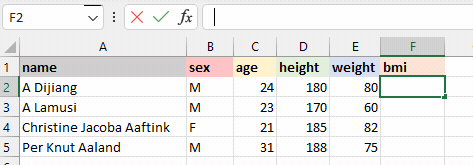We can also add the Excel formula by using assisted GUI. It is simple.

In the example below, we will be using GUI to add an IF formula to convert ‘M’ to ‘Male’ and ‘F’ to Female.

1. Click on the fx button next to the formula bar.
2. It will pop up in the window with the most used function.
3. You can either search for the specific formula or select the formula by scrolling. In our case, we will be specifying the IF formula.
4. Add the logic B2=’M” into the logical_test argument.
5. Add “Male” in value_if_true argument and “Female” in value_if_false argument.

The formula works similarly to the if else statement. If the logical_test statement is TRUE, the formula will return “Male” otherwise “Female.”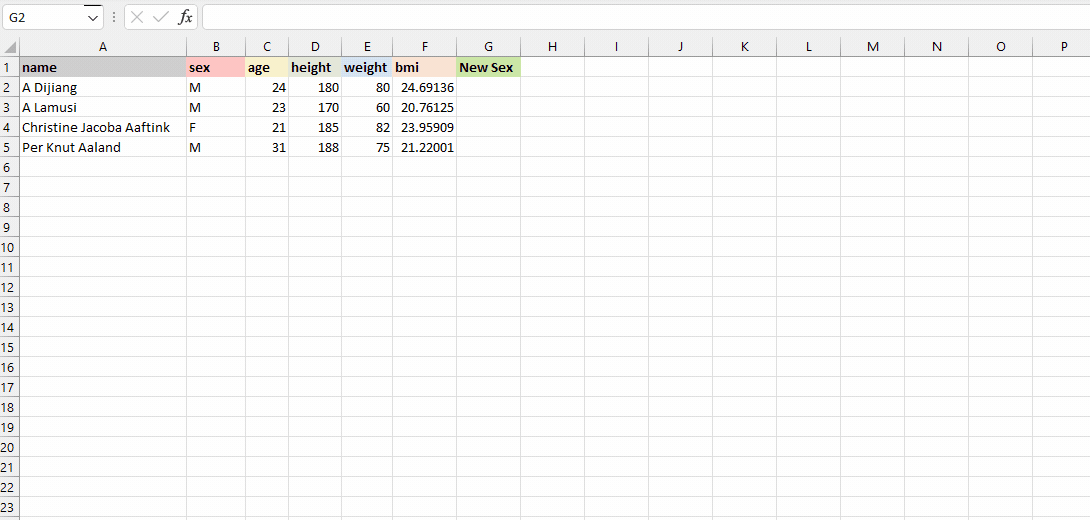Excel formula using UI | Author

### How to insert formulas in excel for an entire column

We have learned to add the formula to a single row. Now, we will learn to apply the same formula to the entire column.

There are multiple ways to add formulas:

• Dragging down the fill handle: when you select the cell, you will see the small green box at the bottom right. It is called a fill handle. Click and hold the fill handle and drag it down to the last row. It is commonly used to apply formulas to selected rows.
• Double click the fill handle: select the cell with the formula and double click the fill handle. Within seconds it will apply the formula to the entire column.
• Shortcut: select the cell with the formula and the empty cells below it. Press CTRL + D to apply the formula. Make sure you are not selecting anything above the formula cell.
• Copy-pasting: copy the cell with the formula (CTRL + C), select the empty rows in a column, and paste it (CTRL + V). Make sure you are not using a fill handle to select the rows.

The visual representation below shows all the ways we can apply the formula to multiple cells.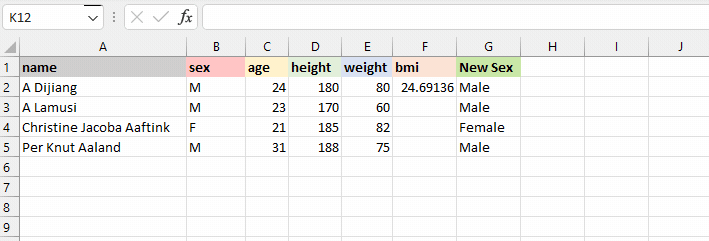Formula for an entire column | Author

## Basic Formulas in Excel

Now that we know how to use Excel formulas, it’s time to learn about 15 basic Excel formulas on a small subset of the Olympics dataset from DataCamp. To keep things simple, we will mainly use the name, sex, age, height, and weight columns of four athletes' records.

### 1. SUM

The SUM() formula performs addition on selected cells. It works on cells containing numerical values and requires two or more cells.

In our case, we will be applying the SUM formula to a range of cells from C2 to C5 and storing the result on C6. It will add 24, 23, 21, and 31. You can also apply this formula to multiple columns.

``=SUM(C2:C5)``

### 2. MIN and MAX

The MIN() formula requires a range of cells, and it returns the minimum value. For example, we want to display the minimum weight among all athletes on the E6 cell. The MIN formula will search for the minimum value and show 60.

``=MIN(E2:E5)``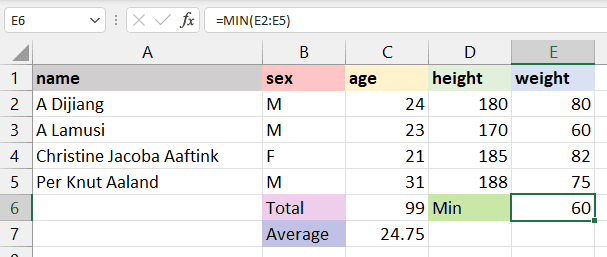The MAX() formula is the opposite of MIN(). It will return the maximum value from the selected range of cells. The formula will look for the maximum value and return 82.

``=MAX(E2:E5)``

### 3. AVERAGE

The AVERAGE() formula calculates the average of selected cells. You can provide a range of cells (C2:C5) or select individual cells (C2, C3, C5).

To calculate the average of athletes, we will select the age column, apply the average formula, and return the result to the C7 cell. It will sum up the total values in the selected cells and divide them by 4.

``=AVERAGE(C2:C5) ``

### 4. COUNT

The COUNT() formula counts the total number of selected cells. It will not count the blank cells and different data formats other than numeric.

We will count the total number of athlete weights, and it will return 4, as we don’t have missing values or strings.

``=COUNT(E2:E5)``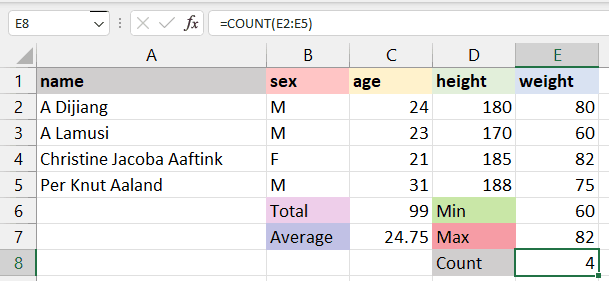To count all types of cells (date-time, string, numerical), you need to use the COUNTA() formula.

The COUNTA formula does not count missing values. For blank cells, use COUNTBLANK().

### 5. POWER

In the beginning, we learned to add power using “^”, which is not an efficient way of applying power to a cell. Instead, we recommended using the POWER() formula to square, cube, or apply any raise to power to your cell.

In our case, we have divided D2 by 100 to get height in meters and squared it by using the POWER formula with the second argument as 2.

``=POWER(D2/100,2)``

### 6. CEILING and FLOOR

The CEILING() formula rounds a number up to the nearest given multiple. In our case, we will round 3.24 up to a multiple of 1 and get 4. If the multiple is 5, it will round up the number 3.24 to 5.

``=CEILING(F2,1)``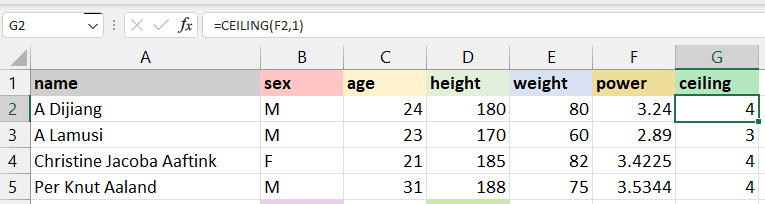The FLOOR() rounds a number down to the nearest given multiple. As we can see in the image below, instead of converting 3.24 to 4, it has rounded the number to 3.

``=FLOOR(F2,1)``

### 7. CONCAT

The CONCAT() Excel formula joins or merges multiple strings or cells with strings into one. For example, if we want to join the age and sex of the athletes, we will use CONCAT. The formula will automatically convert a numeric value from age to string and combine it.

“24”+“M” = “24M”

``=CONCAT(C2,B2)``

### 8. TRIM

TRIM is used to remove extra spaces from the start, middle, and end. It is commonly used to identify duplicate values in cells, and for some reason, extra space makes it unique.

For example:

1. There are extra two spaces at A3 “A   Lamusi”, and it has been successfully removed by TRIM.
2. At A4 “ Christie Jacoba Aaftink”, there is extra space at the start, and without writing any complex formula, the TRIM has removed it.
``=TRIM(A4)``

### 9. REPLACE and SUBSTITUTE

REPLACE is used for replacing part of the string with a new string.

REPLACE(old_text, start_num, num_chars, new_text)

• old_text is the original text or cell containing the text.
• start_num is the index position that you want to start replacing the character.
• num_chars refers to the number of characters you want to replace.
• new_text indicates the new text that you want to replace with old text.

For example, we will change A Dijiang with B Dijiang by providing the positing of character, which is 1, the number of characters that we want to replace, which is also 1, and the new character “B”.

``=REPLACE(A2,1,1,"B")``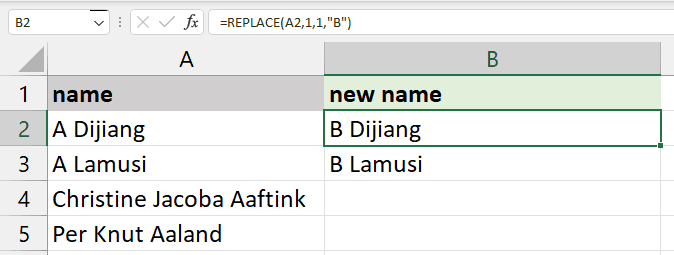The SUBSTITUTE formula is similar to REPLACE. Instead of providing the location of a character or the number of characters, we will only provide old text and new text.

SUBSTITUTE(text, old_text, new_text, [instance_num])

In our case, we are replacing "Jacoba" with "Rahim" to display the result on A4 cell “Christine Rahim Aaftink.”

This formula is quite useful as it does not change the text without “Jacoba” as shown below in cell A5, “Per Knut Aaland.” Whereas, REPLACE will replace the text every time.

``=SUBSTITUTE(A4,"Jacoba","Rahim")``

### 10. LEFT, RIGHT, and MID

The LEFT returns the number of characters from the start of the string or text.

For example, to display the first name from the text “Christine Jacoba Aaftink”, you will use LEFT with 9  numbers of characters. As a result, it will show the first nine characters; “Christine.”

``=LEFT(A2,9)``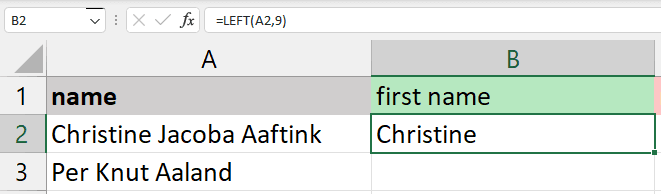The MID formula requires starting position and length to extract the characters from the middle.

For example, if you want to display a middle name, you will start with “J” which is at the 11th position, and 6 for the length of the middle name “Jacoba”.

``=MID(A2,11,6)``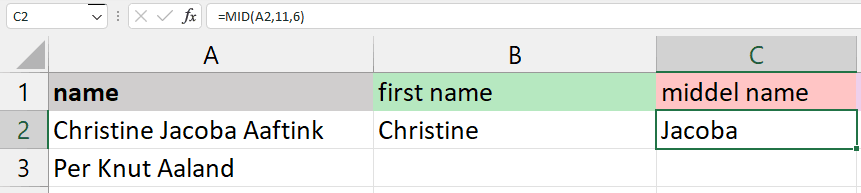The RIGHT will return the number of characters from the end. You just need to provide a number of characters.

For example, to display the last name “Aaftink,” we will use RIGHT with seven characters.

``=RIGHT(A2,7)``

### 11. UPPER, LOWER, and PROPER

The UPPER, LOWER, and PROPER are basic string operations. You can find similar in Tableau or in Python. These formulas only require a text, the location of the cell containing string, or the range of cells with string.

UPPER will convert all the letters in the text to uppercase.

``=UPPER(A1:F1)``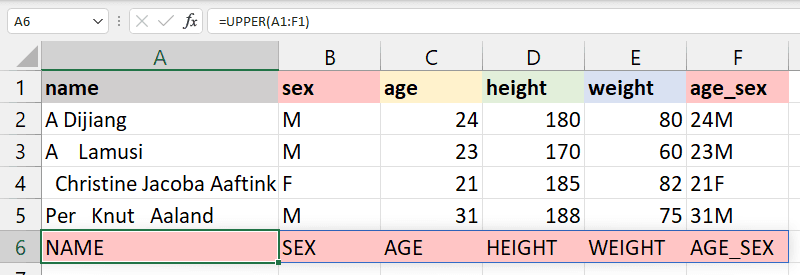LOWER will convert the selected text lower case.

``=LOWER(A1:F1)``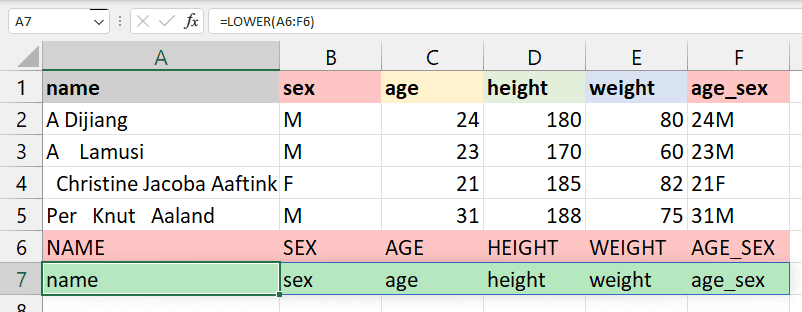PROPER will convert the string to the proper case. For example, the first letter in each word will be capitalized, and the rest of them will be lowercase.

``=PROPER(A1:F1)``

### 12. NOW and TODAY

NOW returns the current time and date, and TODAY returns only the current date. These are quite simple, and we will use them to extract a day, month, year, hours, and minutes from any date time data cell.

The example below returns the current date and time.

``=NOW()``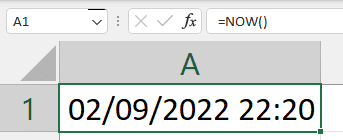To extract the seconds from the time, you will use the SECOND() formula.

``=SECOND(NOW())``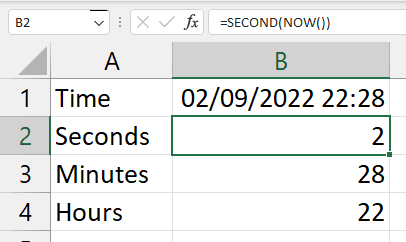Similarly, TODAY will return only the current date.

``=TODAY()``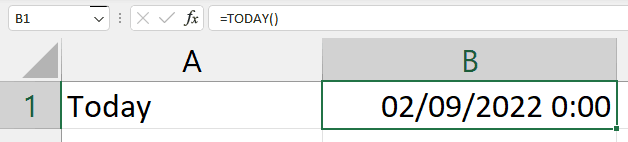To extract the day, you will use the DAY() formula.

Furthermore, you can extract month, year, weekday, day names, hours, and minutes from the date time data field.

``=DAY(TODAY())``

### 13. DATEDIF

It is the most used formula for time series data sets. The DATEDIF calculates the difference between two dates and returns the number of days, months, weeks, or years based on your preference.

In the example below, we want to return the date difference in days by providing “d” for unit arguments. Make sure that the first argument is the start date and the second argument in the formula is the end date.

start_date < end_date

``=DATEDIF(A2,B2,"d")``

### 14. VLOOKUP and HLOOKUP

The worksheet1 that we will use in this section contains all the data from the Olympics dataset.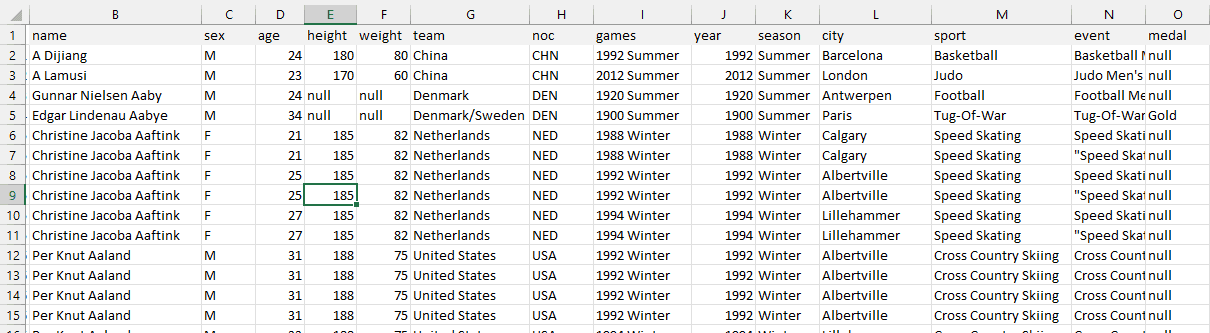worksheet1

The VLOOKUP formula searches for the value in the leftmost column of the table array and returns the value from the same row from the specified columns.

VLOOKUP(lookup_value, table_array, col_index, range_lookup)

• lookup_value: the value you are looking for that is present in the first column.
• table_array: the range of the table, worksheet, or selected cell with multiple columns.
• col_index: the position of the column to extract the value.
• range_lookup: “True” is used for the approximate match (default), and “FALSE” is used for the exact match.

In our case, we are looking for A Dijiang (A2) from selected columns and rows of worksheet1 (B2:H20). The VLOOKUP formula will check the name column in worksheet one and return the 6th column value that is team “China”.

``=VLOOKUP(A2,worksheet1!B2:H20,6,FALSE)``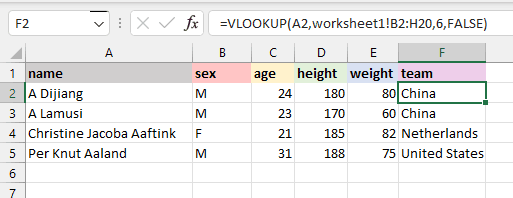The HLOOKUP searches for the value in the first row instead of the first column. It returns the value from the same column and the row you specified.

HLOOKUP(lookup_value, table_array, row_index, range_lookup)

In our case, we will display A Dijaing’s sex on the D8 cell. The HLOOKUP formula will look for the name in the first row and return the value “M'' from the 2nd row of the same column. The range_lookup is kept FALSE in both cases for the exact match.

``=HLOOKUP(B1,B1;E5,2,FALSE)``

### 15. IF

The IF Excel formula is straightforward. It is similar to an if-else statement in a programming language. We will provide the logic of the formula. If the logic is correct, it will return a certain value; if the logic is False, it will return a different value.

For example, if the BMI of athletics is less than 23.9, the formula will return the string “Fit”, else “Unfit”. It is quite useful to convert numerical values into categories.

``=IF(G2<24.9,"Fit,"Unfit")``

## Conclusion

Microsoft Excel is a powerful and easy-to-use application for data analysis and reporting. In this post, we have learned the importance of basic Excel formulas and how they provide us extra ability to perform complex calculations. Furthermore, we have learned about various ways of adding formulas to worksheets and looked in detail at the essential basic Excel formulas.

To learn more about Excel and how to use these formulas for data analysis, take our Data Analysis in Excel course. It will teach you the basics of data exploration, processing, and analysis. If you are fond of the Google stack and use Google Sheets, try Data Analysis in Spreadsheet.

You can also take another step forward by learning the fundamentals of a spreadsheet. You will learn about data analysis, advanced data manipulation techniques, pivot tables, and data visualization.

Beginner
2 hr
5.6K
Bring your spreadsheets to life by mastering fundamental skills such as formulas, operations, and cell references.
See Details

Beginner
4 hr
42.7K
Expand your spreadsheets vocabulary by diving deeper into data types, including numeric data, logical data, and missing data.

### Data Analysis in Excel

Beginner
4 hr
146.1K
Build real-world Excel skills in just 4 hours. This course will show you time-saving shortcuts and essential functions.
See More
Related

### Excel Basics Cheat Sheet

Learn the basics of Excel with our quick and easy cheat sheet. Have the basics of formulas, operators, math functions and more at your fingertips.

Richie Cotton

18 min

### Data Manipulation in Excel Cheat Sheet

Discover how to manipulate data in Excel using this convenient cheat sheet!

### Time Series Analysis with Spreadsheets Tutorial

In this tutorial, you'll learn basic time-series concepts and basic methods for forecasting time series data using spreadsheets.Avinash Navlani

9 min

### Markov Analysis in Spreadsheets Tutorial

Learn Markov Analysis, their terminologies, examples, and perform it in Spreadsheets!Avinash Navlani

9 min